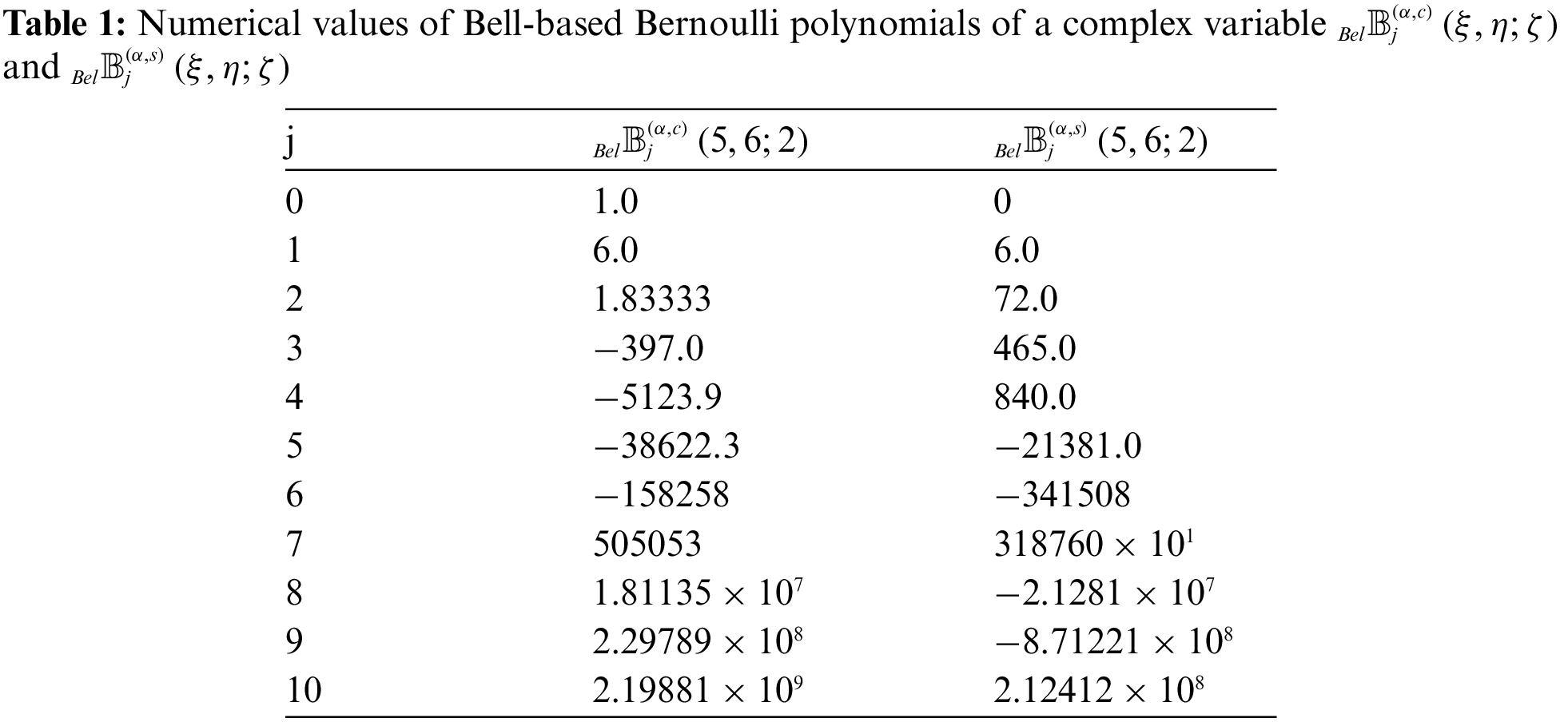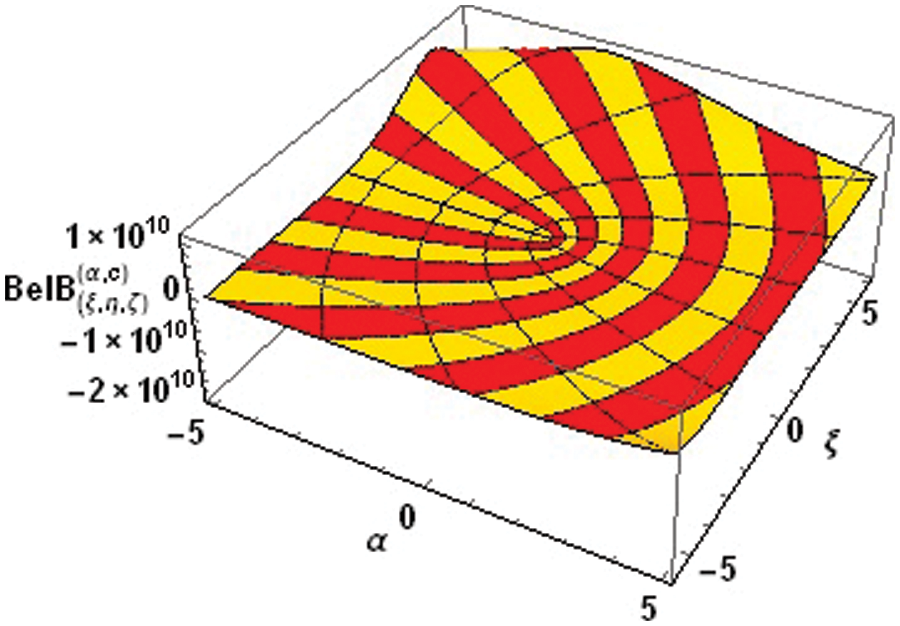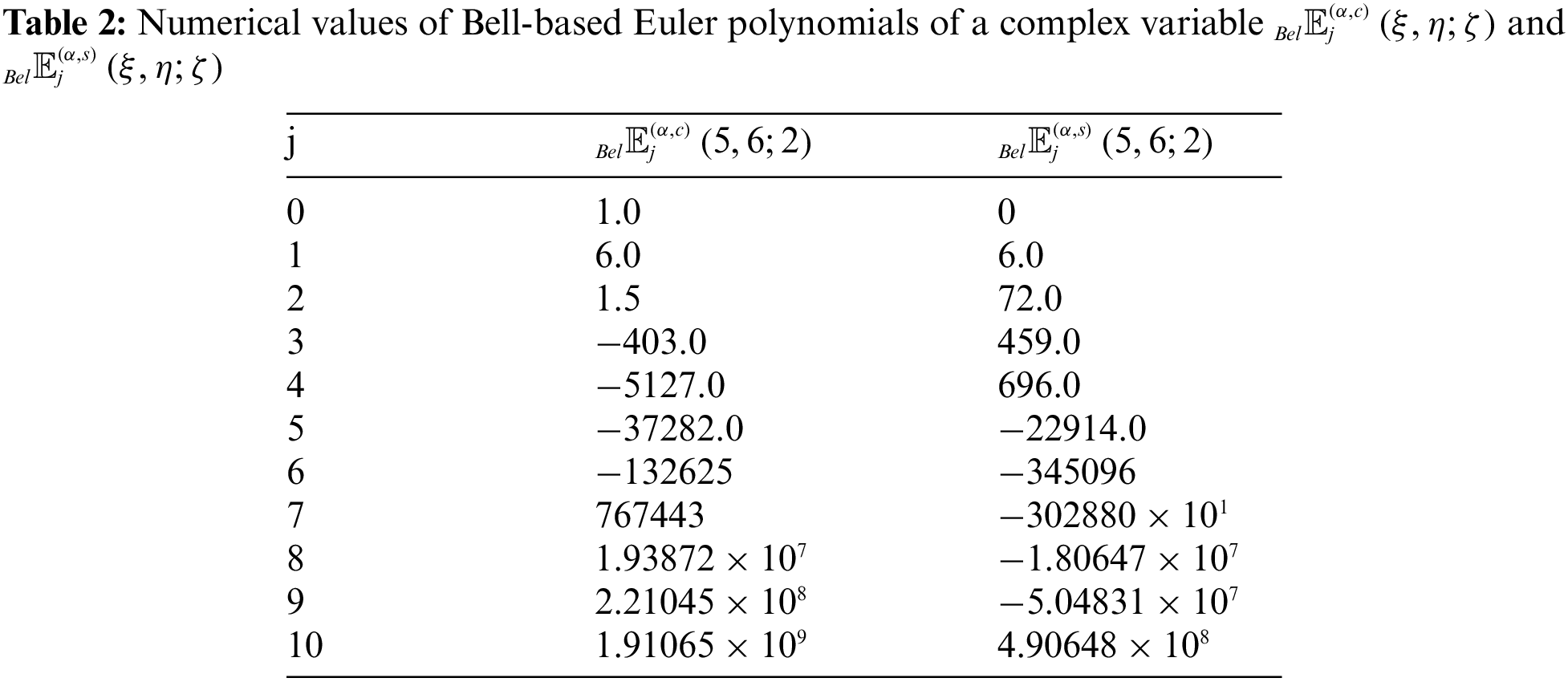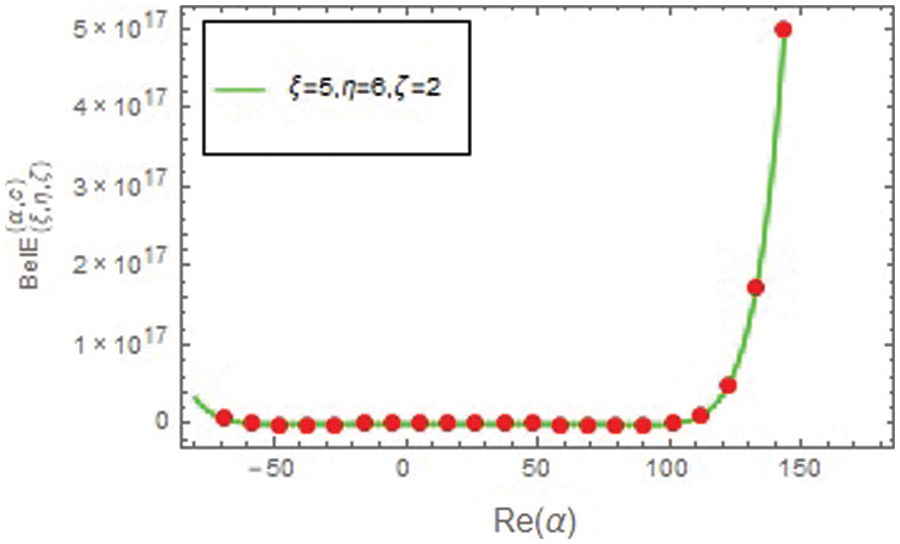Table of ContentOpen Access

ARTICLE

# A Note on Bell-Based Bernoulli and Euler Polynomials of Complex Variable

N. Alam1, W. A. Khan2,*, S. Obeidat1, G. Muhiuddin3, N. S. Diab1, H. N. Zaidi1, A. Altaleb1, L. Bachioua1
1 Department of Basic Sciences, Deanship of Preparatory Year, University of Ha’il, Ha’il, 2440, Saudi Arabia
2 Department of Mathematics and Natural Sciences, Prince Mohammad Bin Fahd University, Al Khobar, 31952, Saudi Arabia
3 Department of Mathematics, Faculty of Science, University of Tabuk, Tabuk, 71491, Saudi Arabia
* Corresponding Author: W. A. Khan. Email:(This article belongs to this Special Issue: Algebra, Number Theory, Combinatorics and Their Applications: Mathematical Theory and Computational Modelling)

Computer Modeling in Engineering & Sciences 2023, 135(1), 187-209. https://doi.org/10.32604/cmes.2022.021418

Received 13 January 2022; Accepted 23 May 2022; Issue published 29 September 2022

### Abstract

In this article, we construct the generating functions for new families of special polynomials including two parametric kinds of Bell-based Bernoulli and Euler polynomials. Some fundamental properties of these functions are given. By using these generating functions and some identities, relations among trigonometric functions and two parametric kinds of Bell-based Bernoulli and Euler polynomials, Stirling numbers are presented. Computational formulae for these polynomials are obtained. Applying a partial derivative operator to these generating functions, some derivative formulae and finite combinatorial sums involving the aforementioned polynomials and numbers are also obtained. In addition, some remarks and observations on these polynomials are given.

### Keywords

Bernoulli polynomials; euler polynomials; bell polynomials; stirling numbers

1  Introduction

Special polynomials and numbers possess much importance in multifarious areas of sciences such as physics, mathematics, applied sciences, engineering and other related research fields covering differential equations, number theory, functional analysis, quantum mechanics, mathematical analysis, mathematical physics and so on (see ) and see also each of the references cited therein. For example, Bernoulli polynomials and numbers are closely related to the Riemann zeta function, which possesses a connection with the distribution of prime numbers. Some of the most significant polynomials in the theory of special polynomials are the Bell, Euler, Bernoulli, Hermite, and Genocchi polynomials. Recently, the aforesaid polynomials and their diverse generalizations have been densely considered and investigated by many physicists and mathematicians (see [118,22]) and see also the references cited therein (see [69,1417]). The class of Appell polynomial sequence is one of the significant classes of polynomials sequence . In applied mathematics, theoretical physics, approximation theory, and several other mathematics branches. The set of Appell polynomial sequence is closed under the operation of umbral composition of polynomial sequences. The Appell polynomial sequence can be given by the following generating function:

A(ξ,z)=A(z)eξz=j=0Aj(ξ)zjj!.

The power series A(z) given by

A(z)=A0+A1z1!+A2z22!++Ajzjj!+=j=0Ajzjj!,(A00),(1)

where Ai{i=1,2,3,} are real coffiecients. It is easy to see that for any A(z), the derivative of A(z) satisfies

ddzAj(z)=jAj1(z),j=0,1,2,3,.

The Bell-based Bernoulli and Bell-based Bernoulli polynomials of the first kind are the special cases of Appell polynomials (see [2,18]).

The generalized Bernoulli and Euler polynomials of order α are defined by (see )

(zez1)αeξz=j=0Bj(α)(ξ)zjj!,z∣<2π,(2)

and

(2ez+1)αeξz=j=0Ej(α)(ξ)zjj!,z∣<π,(3)

respectively.

At the point ξ=0, Bj(α)=Bj(α)(0) and Ej(α)=Ej(α)(0) are the Bernoulli and Euler numbers of order α.

For j0, Stirling numbers of the first kind are defined by

(ξ)j=r=0jS1(j,r)ξr,(see),(4)

where (ξ)0=1, and (ξ)j=ξ(ξ1)(ξj+1),(ξ1). From(4), we see that

1k!(log(1+z))k=j=kS1(j,k)zjj!,(k0),(see).(5)

The Stirling numbers of the second kind are defined by

ξj=r=0jS2(j,r)(ξ)r,(see[12,14,15]).(6)

By (5), we note that

1r!(ez1)r=j=rS2(j,r)zjj!,(see).(7)

The generating function of Bell polynomials Belj(ξ) are defined by (see )

eξ(ez1)=j=0Belj(ξ)zjj!.(8)

In the special case ξ=1, Belj=Belj(1),(j0) are the Bell numbers. From (1.7) and (1.8), we have

Belj(ξ)=r=0jS2(j,r)ξr,(j0).(9)

Recently, Duran et al.  introduced the generalized Bell-based Bernoulli polynomials are defined by

(zez1)αeξz+η(ez1)=j=0BelBj(α)(ξ;η)zjj!.(10)

At the point ξ=η=1, BelBj(α)=BelBj(α)(1;1) are the generalized Bell-based Bernoulli numbers.

Kim et al.  and Jamei et al. [4,5] introduced the Bernoulli and Euler polynomials of complex variable are defined by

zez1eξzcosηz=j=0Bj(ξ+iη)+Bj(ξiη)2zjj!=j=0Bj(c)(ξ,η)zjj!,(11)

zez1eξzsinηz=j=0Bj(ξ+iη)Bj(ξiη)2izjj!=j=0Bj(s)(ξ,η)zjj!,(12)

and

2ez+1eξzcosηz=j=0Ej(ξ+iη)+Ej(ξiη)2zjj!=j=0Ej(c)(ξ,η)zjj!,(13)

2ez+1eξzsinηz=j=0Ej(ξ+iη)Ej(ξiη)2izjj!=j=0Ej(s)(ξ,η)zjj!,(14)

respectively.

Also they have prove that (see [4,5,8,9,19,20,21])

eξzcosηz=r=0Cr(ξ,η)zrr!,(15)

and

eξzsinηz=r=0Sr(ξ,η)zrr!,(16)

where

Cr(ξ,η)=j=0[r2](1)j(r2j)ξr2jη2j,(17)

and

Sr(ξ,η)=j=0[r12](r2j+1)(1)jξr2j1η2j+1.(18)

Motivated by the importance and potential applications in certain problems in number theory, combinatorics, classical and numerical analysis and physics, several families of Bernoulli and Euler polynomials and special polynomials have been recently studied by many authors, see [8,9,1921]. Recently, Kim et al. [13,16] have introduced the degenerate Bernoulli and degenerate Euler polynomials of a complex variable. By separating the real and imaginary parts, they introduced the parametric kinds of these degenerate polynomials. The manuscript of this paper is arranged as follows. In Section 2, we introduce parametric kinds of Bell-based Bernoulli polynomials and prove several identities of Bell-based Bernoulli polynomials by using different analytical means and applying generating functions. In Section 3, we establish parametric kinds of Bell-based Euler polynomials and investigate some identities of these polynomials.

2  Bell-Based Bernoulli Polynomials of Complex Variable

In this section, we consider the Bell-based Bernoulli polynomials of complex variable and deduce some identities of these polynomials. First, we present the following definition as

(zez1)αe(ξ+iη)zeζ(ez1)=j=0BelBj(α)(ξ+iη;ζ)zjj!.(19)

On the other hand, we suppose that

e(ξ+iη)z=eξzeiηz=eξz(cosηz+isinηz).(20)

Thus, by (19) and (20), we have

j=0BelBj(α)(ξ+iη;ζ)zjj!=(zez1)αe(ξ+iη)zeζ(ez1)=(zez1)αeξz(cosηz+isinηz)eζ(ez1),(21)

and

j=0BelBj(α)(ξiη;ζ)zjj!=(zez1)αe(ξiη)zeζ(ez1)=(zez1)αeξz(cosηzisinηz)eζ(ez1).(22)

From (21) and (22), we get

(zez1)αeξzcosηzeζ(ez1)=j=0(BelBj(α)(ξ+iη;ζ)+BelBj(α)(ξiη;ζ)2)zjj!,(23)

and

(zez1)αeξzsinηzeζ(ez1)=j=0(BelBj(α)(ξ+iη,ζ)BelBj(α)(ξiη;ζ)2i)zjj!.(24)

Definition 2.1. Let j0. We define two parametric kinds of cosine Bell-based Bernoulli polynomials BelBj(α,c)(ξ,η;ζ) and sine Bell-based Bernoulli polynomials BelBj(α,s)(ξ,η;ζ), for non negative integer j are defined by

(zez1)αeξzcosηzeζ(ez1)=j=0BelBj(α,c)(ξ,η;ζ)zjj!,(25)

and

(zez1)αeξzsinηzeζ(ez1)=j=0BelBj(α,s)(ξ,η;ζ)zjj!,(26)

respectively.

Note that BelBj(α,c)(ξ,0;0)=Bj(α)(ξ),BelBj(α,s)(0,0;0)=0,(j0).

From (23)(26), we have

BelBj(α,c)(ξ,η;ζ)=BelBj(α)(ξ+iη;ζ)+BelBj(α)(ξiη;ζ)2,(27)

BelBj(α,s)(ξ,η;ζ)=BelBj(α)(ξ+iη;ζ)BelBj(α)(ξiη;ζ)2i.(28)

Remark 2.1. For ξ=ζ=0 in (25) and (26), we get

(zez1)αcosηz=j=0Bj(α,c)(η)zjj!,(29)

and

(zez1)αsinηz=j=0Bj(α,s)(η)zjj!,(30)

respectively.

It is clear that

Bj(α,c)(0)=Bj(α,c),Bj(α,s)(0)=0,(j0).

Remark 2.2. Letting ζ=0 in (25) and (26), we obtain

(zez1)αeξzcosηz=j=0Bj(α,c)(ξ;η)zjj!,(31)

and

(zez1)αeξzsinηz=j=0Bj(α,s)(ξ;η)zjj!,(32)

respectively.

Remark 2.3. On setting ξ=0 in (25) and (26), we acquire

(zez1)αcosηzeζ(ez1)=j=0BelBj(α,c)(η;ζ)zjj!,(33)

and

(zez1)αsinηzeζ(ez1)=j=0BelBj(α,s)(η;ζ)zjj!,(34)

respectively.

Now, we start some basic properties of these polynomials.

Theorem 2.1. Let j0. Then

BelBj(α,c)(η;ζ)=v=0[j2](j2v)(1)vη2vBelBj2v(α)(ζ),(35)

and

BelBj(α,s)(η;ζ)=v=0[j12](j2v+1)(1)vη2v+1BelBj2v1(α)(ζ).(36)

Proof. By (33) and (34), we can derive the following equations:

j=0BelBj(α,c)(η;ζ)zjj!=(zez1)αcosηzeζ(ez1)=j=0BelBj(α,c)(ζ)zjj!v=0(1)vη2vzv2v!.=j=0(v=0[j2](j2v)(1)vη2vBelBj2v(α)(ζ))zjj!,(37)

and

j=0BelBj(α,s)(η;ζ)zjj!=(zez1)αsinηzeζ(ez1)=j=0(v=0[j12](j2v+1)(1)vη2v+1BelBj2v1(α)(ζ))zjj!.(38)

Therefore, by (37) and (38), we get (35). Similarly, we can easily obtain (36).

Theorem 2.2. Let j0. Then

BelBj(α)(ξ+iη;ζ)=u=0j(ju)(ξ+iη)juBelBu(α)(ζ)=u=0j(ju)(iη)juBelBu(α)(ξ;ζ),(39)

and

BelBj(α)(ξiη;ζ)=u=0j(ju)(ξiη)juBelBu(α)(ζ)=u=0j(ju)(1)ju(iη)juBelBu(α)(ξ;ζ).(40)

Proof. By using (21) and (22), we obtain (39) and (40). So we omit the proof.

Theorem 2.3. Let j0. Then

BelBj(α,c)(ξ,η;ζ)=u=0j(ju)BelBju(α)(ζ)Cu(ξ,η),(41)

and

BelBj(α,s)(ξ,η;ζ)=u=0j(ju)BelBju(α)(ζ)Su(ξ,η).(42)

Proof. Consider

(j=0ajzjj!)(v=0bvzvv!)=j=0(v=0jajvbv)zjj!.

Now

j=0BelBj(α,c)(ξ,η,ζ)zjj!=(zez1)αeξzcosηzeζ(ez1)

=(j=0BelBj(α)(ζ)zjj!)(v=0Cv(ξ,η)zjj!)

=j=0(v=0j(jv)BelBjv(α)(ζ)Cv(ξ,η))zjj!,

which proves (41). The proof of (42) is similar.

Theorem 2.4. For every jZ+, we have

BelBj(α,c)(ξ,η;ζ)=k=0jBk(α,c)(ξ;η)Beljk(ζ),(43)

BelBj(α,s)(ξ,η;ζ)=k=0jBk(α,s)(ξ;η)Beljk(ζ),(44)

BelBj(α,c)(ξ,η;ζ)=k=0jBelBk(α,c)(η;ζ)ξjk,(45)

and

BelBj(α,s)(ξ,η;ζ)=k=0jBelBk(α,s)(η;ζ)ξjk.(46)

Proof. Using (25) and (26), we obtain (43)(46). Here, we omit the proof of the theorem.

Theorem 2.5. Let j0. Then

BelBj(α,c)(ξ+s,η;ζ)=u=0j(ju)BelBu(α,c)(ξ,η,ζ)sju,(47)

and

BelBj(α,s)(ξ+s,η;ζ)=u=0j(ju)BelBu(α,s)(ξ,η,ζ)sju.(48)

Proof. By changing ξ with ξ+s in (25), we have

j=0BelBj(α,c)(ξ+s,η;ζ)zjj!=(zez1)αeξzcosηzeszeζ(ez1)=(j=0BelBj(α,c)(ξ,η;ζ)zjj!)(u=0suzuu!)=j=0(u=0j(ju)BelBu(α,c)(ξ,η;ζ)sju)zjj!,

which complete the proof (47). The result (48) can be similarly proved.

Theorem 2.6. Let j1. Then

ξBelBj(α,c)(ξ,η;ζ)=jBelBj1(α,c)(ξ,η;ζ),(49)

ηBelBj(α,c)(ξ,η;ζ)=jBelBj1(α,s)(ξ,η;ζ),(50)

and

ξBelBj(α,s)(ξ,η;ζ)=jBelBj1(α,s)(ξ,η;ζ),(51)

ηBelBj(α,s)(ξ,η;ζ)=jBelBj1(α,c)(ξ,η;ζ).(52)

Proof. Eq. (25) yields

j=1ξBelBj(α,c)(ξ,η;ζ)zjj!=(zez+1)αzeξzcosηzeζ(ez1)=j=0BelBj(α,c)(ξ,η;ζ)zj+1j!=j=1BelBj1(α,c)(ξ,η;ζ)zj(j1)!=j=1jBelBj1(α,c)(ξ,η;ζ)zjj!,

proving (49). Other (50), (51) and (52) can be similarly derived.

Theorem 2.7. Let j1. Then

ζBelBj(α,c)(ξ,η;ζ)=BelBj1(α1,c)(ξ,η;ζ),(53)

and

ζBelBj(α,s)(ξ,η;ζ)=BelBj1(α1,s)(ξ,η;ζ).(54)

Proof. By (25), we have

ζj=1BelBj(α,c)(ξ,η;ζ)zjj!=ζ(zez1)αeξzcosηzeζ(ez1)=(zez1)αeξzcosηzζeζ(ez1)=(zez1)αeξzcosηz(ez1)eζ(ez1)=(zez1)α1eξzcosηzeζ(ez1)z=j=1BelBj1(α1,c)(ξ,η;ζ)zjj!.(55)

The complete proof of (53). The proof of (54) is similar.

Theorem 2.8. For j0. Then

Belj(c)(ξ,η;ζ)=BelBj(c)(ξ+1,η;ζ)BelBj(c)(ξ,η;ζ)j+1(56)

, and

Belj(s)(ξ,η;ζ)=BelBj(s)(ξ+1,η,ζ)BelBj(s)(ξ,η;ζ)j+1.(57)

Proof. By (25), we have

eξzcosηzeζ(ez1)=ez1zj=0BelBj(c)(ξ,η;ζ)zjj!=1z[j=0BelBj(c)(ξ+1,η;ζ)zjj!j=0BelBj(c)(ξ,η;ζ)zjj!]=j=0BelBj(c)(ξ+1,η,ζ)BelBj(c)(ξ,η;ζ)j+1zjj!.(58)

On the other hand, we have

eξzcosηzeζ(ez1)=j=0Belj(c)(ξ,η;ζ)zjj!.(59)

In view of (58) and (59), we get (56). Similarly, we can easily obtain (57).

Theorem 2.9. Let j0. Then

Belj(c)(ξ,η;ζ)=j!α!(j+α)!m=0j+αα=0m(j+αm)S2(m,α)BelBj+αm(α,c)(ξ,η;ζ),(60)

and

Belj(s)(ξ,η;ζ)=j!α!(j+α)!m=0j+αα=0m(j+αm)S2(m,α)BelBj+αm(α,s)(ξ,η;ζ).(61)

Proof. In definition 2.1, we have

eξzcosηzeζ(ez1)=α!(ez1)αα!j=0BelBj(α,c)(ξ,η;ζ)zjj!=α!zαα=mS2(m,α)zmm!j=0BelBj(α,c)(ξ,η;ζ)zjj!=α!zαm=0α=0mS2(m,α)zmm!j=0BelBj(α,c)(ξ,η;ζ)zjj!=α!j=0m=0j+αα=0m(j+αm)S2(m,α)BelBj+αm(α,c)(ξ,η;ζ)zj(j+α)!.(62)

On the other hand, we have

eξzcosηzeζ(ez1)=j=0Belj(c)(ξ,η;ζ)zjj!.(63)

Therefore, by (62) and (63), we obtain (60). Similarly, we can easily obtain (61).

Theorem 2.10. Let j0. Then

BelBj(α,c)(ξ,η;ζ)=u=0jk=0u(ju)BelBju(α,c)(η;ζ)(ξ)kS2(u,k),(64)

and

BelBj(α,s)(ξ,η;ζ)=u=0jk=0u(ju)BelBju(α,s)(η;ζ)(ξ)kS2(u,k).(65)

Proof. Using (7) and (25), we find

j=0BelBj(α,c)(ξ,η;ζ)zjj!=(zez1)αcosηzeζ(ez1)(ez1+1)ξ=(zez1)αcosηzeζ(ez1)k=0(ξ)k(ez1)kk!=(zez1)αcosηzeζ(ez1)k=0(ξ)ku=kS2(u,k)zuu!=j=0BellBj(α,c)(η;ζ)zjj!u=0k=0u(ξ)kS2(u,k)zuu!=j=0u=0jk=0u(ju)BelBju(α,c)(η;ζ)(ξ)kS2(u,k)zjj!.(66)

In view of (25) and (66), we get (64). Similarly, we can easily obtain (65).

3  Bell-Based Euler Polynomials of Complex Variable

In this section, we define Bell-based Euler polynomials of complex variable and derive some explicit expressions of these polynomials. Now we start with the following definition as

(2ez+1)αe(ξ+iη)teζ(ez1)=j=0BelEj(α)(ξ+iη;ζ)zjj!.(67)

By using (67) and (20), we have

j=0BelEj(α)(ξ+iη;ζ)zjj!=(2ez+1)αe(ξ+iη)zeζ(ez1)=(2ez+1)αeξz(cosηz+isinηz)eζ(ez1),(68)

and

j=0BelEj(α)(ξiη;ζ)zjj!=(2ez+1)αe(ξiη)teζ(ez1)=(2ez+1)αeξz(cosηzisinηz)eζ(ez1).(69)

From (68) and (69), we get

(2ez+1)αeξzcosηzeζ(ez1)=j=0(BelEj(α)(ξ+iη;ζ)+BelEj(α)(ξiη;ζ)2)zjj!,(70)

and

(2ez+1)αeξzsinηzeζ(ez1)=j=0(BelEj(k)(ξ+iη;ζ)BelEj(k)(ξiη;ζ)2i)zjj!.(71)

Definition 3.1. Let j0. We define two parametric kinds of cosine Bell-based Euler polynomials BelEj(α,c)(ξ,η;ζ) and sine Bell-based Euler polynomials BelEj(α,s)(ξ,η;ζ), for non negative integer j are defined by

(2ez+1)αeξzcosηzeζ(ez1)=j=0BelEj(α,c)(ξ,η;ζ)zjj!,(72)

and

(2ez+1)αeξzsinηzeζ(ez1)=j=0BelEj(α,s)(ξ,η;ζ)zjj!,(73)

respectively.

From (70)(73), we have

BelEj(α,c)(ξ,η;ζ)=BelEj(α)(ξ+iη;ζ)+BelEj(α)(ξiη;ζ)2,

BelEj(α,s)(ξ,η;ζ)=BelEj(k)(ξ+iη;ζ)BelEj(α)(ξiη;ζ)2i.

Note that

BelEj(α,c)(ξ,0,0)=Ej(α)(ξ),BelEj(α,s)(ξ,0,0)=0,(j0).

Remark 3.1. For ξ=0 in (72) and (73), we get

(2ez+1)αcosηzeζ(ez1)=j=0BelEj(α,c)(η;ζ)zjj!,(74)

and

(2ez+1)αsinηzeζ(ez1)=j=0BelEj(α,s)(η;ζ)zjj!,(75)

respectively.

Remark 3.2. Letting ζ=0 in (72) and (73), we obtain

(2ez+1)αeξzcosηz=j=0Ej(α,c)(η;ζ)zjj!,(76)

and

(2ez+1)αeξzsinηz=j=0Ej(α,s)(η;ζ)zjj!,(77)

respectively.

Remark 3.3. On taking ξ=ζ=0 in (72) and (73), we acquire

(2ez+1)αcosηz=j=0Ej(α,c)(η)zjj!,(78)

and

(2ez+1)αsinηz=j=0Ej(α,s)(η)zjj!,(79)

respectively.

Theorem 3.1. Let j0. Then

BelEj(α,c)(η;ζ)=m=0[j2](j2m)(1)mη2mBelEj2m(α)(ζ),(80)

and

BelEj(α,s)(η;ζ)=m=0[j12](j2m+1)(1)mη2m+1BelEj2m1(α)(ζ).(81)

Proof. From (78) and (79), we can derive the following equations:

j=0BelEj(α,c)(η;ζ)zjj!=(2ez+1)αcosηzeζ(ez1)=j=0BelEj(α,c)(ζ)zjj!j=0(1)mζ2mzm2m!.=j=0(m=0[j2](j2m)(1)mη2mBelEj2m(α)(η))zjj!,(82)

and

j=0BelEj(α,s)(η;ζ)zjj!=(2ez+1)αsinηzeζ(ez1)=j=0(m=0[j12](j2m+1)(1)mη2m+1BelEj2m1(α)(ζ))zjj!.(83)

Therefore, by (82) and (83), we get (80). Similarly, we can easily obtain (81).

Theorem 3.2. Let j0. Then

BelEj(α)(ξ+iη;ζ)=l=0j(jl)(ξ+iη)jlBelEl(α)(ζ)=l=0j(jl)(iη)jlBelEl(α)(ξ,ζ),(84)

and

BelEj(α)(ξiη;ζ)=l=0j(jl)(ξiη)jlBelEl(α)(ζ)=l=0j(jl)(1)jl(iη)jlBelEl(α)(ξ,ζ).(85)

Proof. By using (68) and (69), we can easily get (84) and (85). So we omit the proof.

Theorem 3.3. Let j0. Then

BelEj(α,c)(ξ,η;ζ)=m=0j(jm)BelEjm(α)(ζ)Cm(ξ,η),(86)

and

BelEj(α,s)(ξ,η;ζ)=m=0j(jm)BelEjm(α)(ζ)Sm(ξ,η).(87)

Proof. Consider the identity, we have

(j=0ajzjj!)(m=0bmzmm!)=j=0(m=0jajmbm)zjj!.(88)

Now

j=0BelEj(α,c)(ξ,η;ζ)zjj!=(2ez+1)αeξzcosηzeζ(ez1)=(j=0BelEj(α)(ζ)zjj!)(m=0Cm(ξ,η)zmm!)=j=0(m=0j(jm)BelEjm(α)(ζ)Cm(ξ,η))zjj!,

which proves (86). The proof of (87) is similar.

Theorem 3.4. Let j0. Then

BelEj(α,c)(ξ,η;ζ)=k=0jEk(α,c)(η;ζ)Beljk(ζ),(88)

BelEj(α,s)(ξ,η;ζ)=k=0jEk(α,s)(η;ζ)Beljk(ζ),(89)

BelEj(α,c)(ξ,η;ζ)=k=0jBelEk(α,c)(η;ζ)ξjk,(89)

and

BelEj(α,s)(ξ,η;ζ)=k=0jBelEk(α,s)(η;ζ)ξjk,(90)

Proof. Using (72) and (73), we obtain (88)(90). Here, we omit the proof of the theorem.

Theorem 3.5. Let j0. Then

BelEj(α,c)(ξ+s,η;ζ)=m=0j(jm)BelEm(α,c)(ξ,η;ζ)sjm,(91)

and

BelEj(α,s)(ξ+r,η;ζ)=m=0j(jm)BelEm(α,s)(ξ,η;ζ)zjm.(92)

Proof. By changing ξ with ξ+s in (72), we have

j=0BelEj(α,c)(ξ+s,η;ζ)zjj!=(2ez+1)αeξzcosηzeszeζ(ez1)=(j=0BelEj(α,c)(ξ,η;ζ)zjj!)(j=0sjzjj!)=j=0(m=0j(jm)BelEm(α,c)(ξ,η;ζ)sjm)zjj!,

which proves (91). The result (92) can be similarly proved.

Theorem 3.6. Let j1. Then

ξBelEj(α,c)(ξ,η;ζ)=jBelEj1(α,c)(ξ,η;ζ),(93)

ηBelEj(α,c)(ξ,η;ζ)=jBelEj1(α,s)(ξ,η;ζ),(94)

and

ξBelEj(α,s)(ξ,η;ζ)=jBelEj1(α,s)(ξ,η;ζ),(95)

ηBelEj(α,s)(ξ,η;ζ)=jBelEj1(α,c)(ξ,η;ζ).(96)

Proof. Eq. (72) yields

j=1BelEj(α,c)(ξ,η;ζ)ξzjj!=(2ez+1)αzeξzcosηzeζ(ez1)=j=0BelEj(α,c)(ξ,η;ζ)zj+1j!=j=1BelEj1(α,c)(ξ,η;ζ)zj(j1)!=j=1 jBelEj1(α,c)(ξ,η;ζ)zjj!,

proving (93). Other (94)(96) can be similarly derived.

Theorem 3.7. Let j0. Then

ζBelEj(α,c)(ξ,η;ζ)=BelEj(α,c)(ξ+1,η;ζ)BelEj(α,c)(ξ,η;ζ),(97)

and

ζBelEj(α,s)(ξ,η;ζ)=BelEj(α,s)(ξ+1,η;ζ)BelEj(α,s)(ξ,η;ζ).(98)

Proof. By definition (72), we have

ζj=0BelEj(α,c)(ξ,η;ζ)zjj!=ζ(2ez+1)αeξzcosηzeζ(ez1)=(2ez+1)αeξzcosηzζeζ(ez1)=(2ez+1)αeξzcosηz(ez1)eζ(ez1)=j=0[BelEj(α,c)(ξ+1,η;ζ)BelEj(α,c)(ξ,η;ζ)]zjj!.(99)

The complete proof of the result (97). The proof of (98) is similar.

Theorem 3.8. Let j0. Then

Belj(c)(ξ,η;ζ)=12[BelEj(c)(ξ+1,η,ζ)+BelEj(c)(ξ,η;ζ)],(100)

and

Belj(s)(ξ,η;ζ)=12[BelEj(s)(ξ+1,η,ζ)+BelEj(s)(ξ,η;ζ)].(101)

Proof. Using definition 3.1, we have

eξzcosηzeζ(ez1)=ez+12j=0BelEj(c)(ξ,η;ζ)zjj!=12[j=0BelEj(c)(ξ+1,η,ζ)zjj!+j=0BelEj(c)ξ,η;ζ)zjj!]=12j=0[BelEj(c)(ξ+1,η;ζ)+BelEj(c)ξ,η;ζ)]zjj!.(102)

On the other hand, we have

eξzcosηzeζ(ez1)=j=0Belj(c)(ξ,η;ζ)zjj!.(103)

In view of (102) and (103), we get (100). Similarly, we can easily obtain (101).

Theorem 3.9. Let j0. Then

BelEj(α,c)(ξ,η;ζ)=l=0jk=0l(jl)BelEjl(α,c)(η;ζ)(ξ)kS2(l,k),(104)

and

BelEj(α,s)(ξ,η;ζ)=l=0jk=0l(jl)BelEjl(α,s)(η;ζ)(ξ)kS2(l,k).(105)

Proof. Using (7) and (72), we find

j=0BelEj(α,c)(ξ,η;ζ)zjj!=(2ez+1)αcosηzeζ(ez1)(ez1+1)ξ=(2ez+1)αcosηzeζ(ez1)k=0(ξ)k(ez1)kk!=(2ez+1)αcosηzeζ(ez1)k=0(ξ)kl=kS2(l,k)zll!=j=0BelEj(α,c)(η;ζ)zjj!l=0k=0l(ξ)kS2(l,k)zll!=j=0l=0jk=0l(jl)BelEjl(α,c)(η;ζ)(ξ)kS2(l,k)zjj!.(106)

In view of (72) and (106), we get (104). Similarly, we can easily obtain (105).

4  Computational Values and Graphical Representations of Bell-Based Bernoulli Polynomials of Complex Variable

In this section, certain zeros of the Bell-based Bernoulli polynomials of complex variable BelBj(α,c)(ξ,η;ζ) and BelBj(α,s)(ξ,η;ζ) and beautifully graphical representations are shown.

The first few of them are

BelB0(α,c)(ξ,η;ζ)=1,

BelB1(α,c)(ξ,η;ζ)=α2+ζ+ξ,

BelB2(α,c)(ξ,η;ζ)=α24+(ζξ112)αη2+(ζ+ξ)2+ζ,

BelB3(α,c)(ξ,η;ζ)=ζ18(α2(ζ+ξ))(α2(4ζ+4ξ+1)α+4(ζ+ξ)2+12(ζη2)),

BelB4(α,c)(ξ,η;ζ)=ζ4+4ξζ3+6ζ3+6ξ2ζ2+12ξζ2+7ζ2+4ξ3ζ+6ξ2ζ2αζ+4ξζ4α(ζ+ξ)ζ+ζ+η4+ξ4+1240α(5α(3(α2)α+1)+2)12(α1)α2(ζ+ξ)+12α(3α1)((ζ+ξ)2+ζ)2α(ζ+ξ)((ζ+ξ)2+ζ)+12η2(3α2+12(ζ+ξ)α+α12((ζ+ξ)2+ζ)),

andBelB0(α,s)(ξ,η;ζ)=0,

BelB1(α,s)(ξ,η;ζ)=η,

BelB2(α,s)(ξ,η;ζ)=2η(α2+ζ+ξ),

BelB3(α,s)(ξ,η;ζ)=14η(3α212α(ζ+ξ)α+12((ζ+ξ)2+ζ)4η2),

BelB4(α,s)(ξ,η;ζ)=4ζη12η(α2(ζ+ξ))(α2α(4ζ+4ξ+1)+4(ζ+ξ)2+12ζ4η2).

Table 1 shows some numerical values of Bell-based Bernoulli polynomials of a complex variable.Fig. 1 shows the plot for the Bell-based Bernoulli polynomial BelBj(α,c)(ξ,η;ζ) with (ξ,η;ζ)=(5,6;2). Fig. 2 shows the plot for 3D Bell-based Bernoulli polynomials BelBj(α,c)(ξ,η;ζ).Figure 1: Bell-based Bernoulli polynomials BelBj(α,c)(ξ,η;ζ)Figure 2: 3D Bell-based Bernoulli polynomials BelBj(α,c)(ξ,η;ζ)

5  Computational Values and Graphical Representations of Bell-Based Euler Polynomials of Complex Variable

In this section, certain zeros of the Bell-based Bernoulli polynomials of complex variable BelEj(α,c)(ξ,η;ζ) and BelEj(α,s)(ξ,η;ζ) and beautifully graphical representations are shown.

The first few of them are

BelE0(α,c)(ξ,η;ζ)=1,

BelE1(α,c)(ξ,η;ζ)=α2+ζ+ξ,

BelE2(α,c)(ξ,η;ζ)=α24+ζη2+(ζ+ξ)214α(1+4ζ+4ξ),

BelE3(α,c)(ξ,η;ζ)=ζ18(α2(ζ+ξ))(α2+12(ζη2))+4(ζ+ξ)2α(3+4ζ+4ξ),

BelE4(α,c)(ξ,η;ζ)=116(α22α3(3+4ζ+4ξ))+3α2(1+8ζ28η2+16ζ(1+ξ)+8ξ(1+ξ))+16(ζ4+η46η2ξ2+ξ4+ξ3(6+4ξ)+ζ(1+2ξ)(16η2+2ξ(1+ξ)))+ζ2(76η2+6ξ(2+ξ))2α(112η2+4(4ζ3+3ζ2(5+4ξ))+ζ(712η2+6ξ(3+2ξ))+ξ(12η2+ξ(3+4ξ))),

andBelE0(α,s)(ξ,η;ζ)=0,

BelE1(α,s)(ξ,η;ζ)=η,

BelE2(α,s)(ξ,η;ζ)=η(α+2(ζ+ξ)),

BelE3(α,s)(ξ,η;ζ)=14η(12ζ4η2+3(α2+4(ζ+ξ)2α(1+4ζ+4ξ))),

BelE4(α,s)(ξ,η;ζ)=12η(8ζ(α2(ζ+ξ))(α2+12ζ4η2)+4(ζ+ξ)2α(3+4ζ+4ξ)).

Table 2 shows some numerical values of Numerical values of Bell-based Euler polynomials of complex variable.Fig. 3 shows the plot for the Bell-based Euler polynomial BelEj(α,c)(ξ,η;ζ) with (ξ,η;ζ)=(5,6;2).Figure 3: Bell-based Euler polynomials BelBj(α,c)(ξ,η;ζ)

6  Conclusions

In the present article, we have considered the parametric kinds of Bell-based Bernoulli and Euler polynomials by making use of the exponential as well as trigonometric functions. We have also derived some analytical properties of our newly introduced parametric polynomials by using the series manipulation technique. Furthermore, it is noticed that, if we consider any Appell polynomials of a complex variable (as discussed in the present article), then we can easily define its parametric kinds by separating the complex variable into real and imaginary parts. Consequently, the results of this article may potentially be used in mathematics, mathematical physics and engineering.

Acknowledgement: The authors wish to express their appreciation to the reviewers for their helpful suggestions which greatly improved the presentation of this paper.

Funding Statement: This research was funded by Research Deanship at the University of Ha’il, Saudi Arabia, through Project No. RG-21 144.

Conflicts of Interest: The authors declare that they have no conflicts of interest to report regarding the present study.

References

1.  1.  Avram, F., Taqqu, M. S. (1987). Noncentral limit theorems and Appell polynomials. The Annals of Probability, 15(2), 767–775. DOI 10.1214/aop/1176992170.
2.  2.  Duran, U., Mehmet, A., Araci, S. (2021). Bell-based Bernoulli polynomials. Axioms, 10(29), 1–23. DOI 10.3390/axioms10010029.
3.  3.  Hussain, S. S., Muhiuddin, G., Durga, N., Al-Kadi, D. (2022). New concepts on quadripartioned bipolar single valued neutrosophic graph. Computer Modeling in Engineering & Sciences, 130(1), 559–580. DOI 10.32604/cmes.2022.017032.
4.  4.  Jamei, M. M., Beyki, M. R., Koepf, W. (2018). A new type of Euler polynomials and numbers. Mediterranean Journal of Mathematics, 15(138), 1–17. DOI 10.1007/s00009-018-1181-1.
5.  5.  Jamei, M. M., Beyki, M. R., Koepf, W. (2017). Symbolic computation of some power trigonometric series. Journal of Symbolic Computation, 80, 273–284. DOI 10.1016/j.jsc.2016.03.004.
6.  6.  Khan, W. A., Muhiuddin, G., Muhyi, A., Al-Kadi, D. (2021). Analytical properties of type 2 degenerate poly-Bernoulli polynomials associated with their applications. Advances in Difference Equations, 2021(1), 420. DOI 10.1186/s13662-021-03575-7.
7.  7.  Kim, D. S., Kim, T. (2015). Some identities of Bell polynomials. Science China Mathematics, 58(10), 1–10. DOI 10.1007/s11425-015-5006-4.
8.  8.  Muhiuddin, G., Khan, W. A., Duran, D., Al-Kadi, D. (2021). Some identities of the multi poly-Bernoulli polynomials of complex variable. Journal of Function Spaces, 2021(2), 1–8. DOI 10.1155/2021/7172054.
9.  9.  Muhiuddin, G., Khan, W. A., Al-Kadi, D. (2021). Construction on the degenerate poly-Frobenius-Euler polynomials of complex variable. Journal of Function Spaces, 2021(1), 1–9. DOI 10.1155/2021/3115424.
10. 10. Muhiuddin, G., Khan, W. A., Muhyi, A., Al-Kadi, D. (2021). Some results on type 2 degenerate poly-Fubini polynomials and numbers. Computer Modeling in Engineering & Sciences, 129(2), 1051–1073. DOI 10.32604/cmes.2021.016546.
11. 11. Muhiuddin, G., Khan, W. A., Younis, J. (2021). Construction of type 2 poly-Changhee polynomials and its applications. Journal of Mathematics, 2021(4), 1–9. DOI 10.1155/2021/7167633.
12. 12. Khan, W. A., Ali, R., Alzobydi, K. A. H., Ahmad, N. (2021). A new family of degenerate poly-Genocchi polynomials with its certain properties. Journal of Function Spaces, 2021(4), 1–8. DOI 10.1155/2021/6660517.
13. 13. Kim, T., Ryoo, C. S. (2018). Some identities for Euler and Bernoulli polynomials and their zeros. Axioms, 7(3), 56. DOI 10.3390/axioms7030056.
14. 14. Kim, T., Khan, W. A., Sharma, S. K., Ghayasuddin, M. (2020). A note on parametric kinds of the degenerate poly-Bernoulli and poly-Genocchi polynomials. Symmetry, 12(4), 614. DOI 10.3390/sym12040614.
15. 15. Kim, T., Ryoo, C. S. (2019). Sheffer type degenerate Euler and Bernoulli polynomials. Filomat, 33(19), 6173–6185. DOI 10.2298/FIL1919173K.
16. 16. Kim, D. S., Kim, T., Lee, H. (2019). A note on degenerate Euler and Bernoulli polynomials of complex variables. Symmetry, 11(9), 1168. DOI 10.3390/sym11091168.
17. 17. Kim, D. S., Kim, T. (2021). Some identities on truncated polynomials associated with degenerate Bell polynomials. Russian Journal of Mathematical Physics, 28(3), 342–355. DOI 10.1134/S1061920821030079.
18. 18. Khan, W. A., Kamarujjama, M., Daud (2022). Construction of partially degenerate Bell-Bernoulli polynomials of the first kind and its certain properties. Analysis. https://www.degruyter.com/journal/key/anly/html?lang=en.
19. 19. Ryoo, C. S., Khan, W. A. (2020). On two bivariate kinds of poly-Bernoulli and poly-Genocchi polynomials. Mathematics, 8(3), 417. DOI 10.3390/math8030417.
20. 20. Sharma, S. K., Khan, W. A., Ryoo, C. S. (2020). A parametric kind of the degenerate Fubini numbers and polynomials. Mathematics, 8(3), 405. DOI 10.3390/math8030405.
21. 21. Sharma, S. K., Khan, W. A., Ryoo, C. S. (2020). A parametric kind of Fubini polynomials of a complex variable. Mathematics, 8, 643. DOI 10.3390/math8040643./1.5114093.
22. 22. Tempesta, P. (2007). Formal groups, Bernoulli type polynomial and L-series. Comptes Rendus Mathematique, 345(6), 303–306. DOI 10.1016/j.crma.2007.05.016.

Alam, N., Khan, W. A., Obeidat, S., Muhiuddin, G., Diab, N. S. et al. (2023). A Note on Bell-Based Bernoulli and Euler Polynomials of Complex Variable. CMES-Computer Modeling in Engineering & Sciences, 135(1), 187–209.This work is licensed under a Creative Commons Attribution 4.0 International License , which permits unrestricted use, distribution, and reproduction in any medium, provided the original work is properly cited.

View

• ### 0

Like

Related articles
• Gökçe Yıldız, Gültekin Tınaztepe,...
• Taekyun Kim, Dae San Kim, Dmitry...
• Lee-Chae Jang, Dae San Kim, Hanyoung...
• Lan Wu, Xue-Yan Chen, Muhammet...
• Cristina B. Corcino, Wilson D....